# Operators in JavaScript

JavaScript Operators: In this tutorial, we are going to learn about the various operators in JavaScript with examples.
Submitted by Siddhant Verma, on October 03, 2019

## JavaScript Operators

Operators just how their name suggests help in carrying out various operations in JavaScript. In this article, we'll see various kinds of operators in JavaScript.

Before starting please open your code editor or if you don't have one please use the below method to use your browser's dev tools to learn JavaScript.

Open the chrome dev console to try out the examples by right-clicking on the browser → selecting inspect → selecting console or simply type f12.

### 1) Arithmetic Operators

Simple arithmetic functions can be performed using arithmetic operators. We have + operator for addition, - operator for subtraction, * operator for multiplication, / operator for division, % operator for remainder/modulus, ++ operator for increment, and -- operator for decrement.

Let's try out some examples,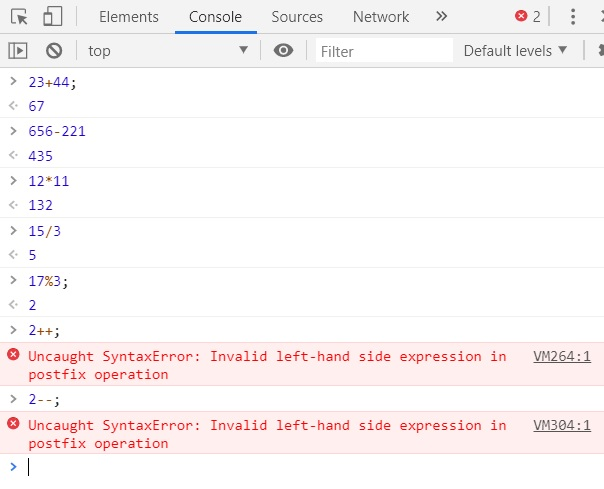For increment and decrement operators, we can only use them with a value stored in a variable. Alternately, we can use all the arithmetic operators on variables with number type.

```var a = 10,
b = 12;
console.log('Value of a: ', a, ' and b: ', b);
console.log(a + b); //22
console.log(a - b); //-2
console.log(a * b); //120
console.log(a / b); //0.8333..
console.log(a % b); //10
console.log(a++); //10
console.log(b--); //12
console.log('New value of a: ', a, ' and b: ', b);
```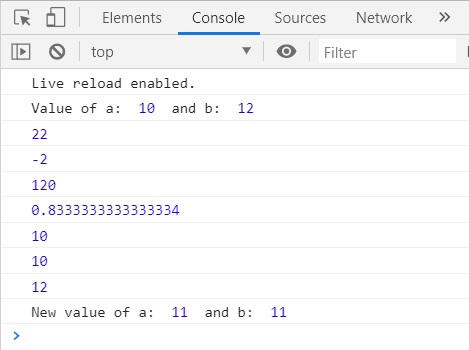Notice when we used the increment and decrement operators we got the original values of a and b while performing the operations on the console. However, later when we output a and b we get the updated values. Why?

This is because we have used a postfix operator that works on the principle of use first then updates. Therefore we get the original value first, values then increments/decrements and then the reference to the new value is made by the same variable.

If we use prefix instead of postfix, we'll get the updated value first because it works on the principle update first then use.

```console.log('Value of a: ',a,' and b: ',b);
console.log(++a); //11
console.log(--b); //11
console.log('New value of a: ',a,' and b: ',b);
```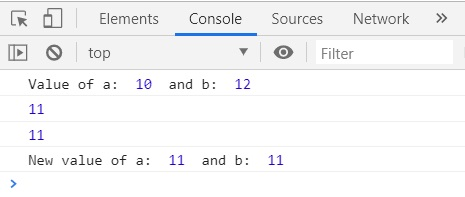### 2) Assignment Operators

The = is used as an assignment operator in JavaScript. It assigns the value on the right-hand side of = to the reference on the left-hand side. Let's try to assign a value to a variable:

```    var a=30;
console.log(a); //30
```

We can also assign the value of another variable to a variable instead of directly assigning the value. This will be useful when you have a dynamic variable that changes during the program and you just want the result to be assigned to some other variable.

```    var a = 30;
var b = a;
console.log(b); //30
```

Now, whatever value takes before being assigned to b will be the same value that b takes.

```var a = 30;

function change(a) {
return (a * 10) + 23;
}

a = change(a)
var b = a;
console.log(b); //323
```

We can use the assignment operator in combination with the arithmetic operators to directly assign the values while carrying out the operation.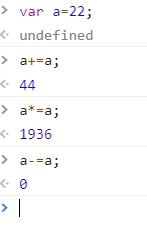a += a means a = a + a. We can use it as a shortcut for carrying out such operations where we want to store the result of an arithmetic operation performed on a variable in the same variable.

When dealing with strings the + operator acts as the concatenation operator. JS understands that adding two strings makes sense only by joining them together. You can also use the += operator to store the concatenation of two strings in the same string.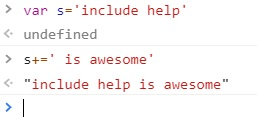### 3) Comparison Operators

For comparing two values, values of two variables or a variable to a value we use the comparison operator.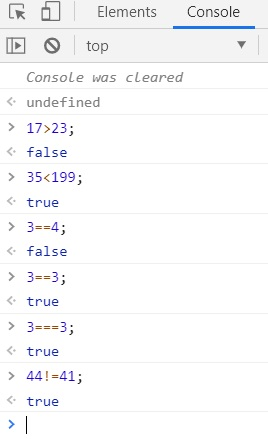These are also called relational operators. Their result evaluates to a boolean. If the comparison made is correct we get a truthy otherwise we get a falsy.

!= checks if two values are not equal and return true, and false otherwise (if they are equal). The > compares for the value on the left-hand side to be greater than the one on the right-hand side and the < compares for the value on the left-hand side to be smaller than the value of the right-hand side. We can combine them with = to get >= or <= operators for handling greater than or equal to and less than or equal to expressions.

Let's look at another examples,

```var thresholdVoltage = 3.4;
var VSS = 2;
var maxVoltage = 5;

function checkCurrent(VSS, thresholdVoltage, maxVoltage) {
if (VSS > thresholdVoltage)
console.log('Current flows');
if (VSS < thresholdVoltage)
console.log('current does not flow');
if (VSS >= maxVoltage)
console.log('Maximum current flows');
}
```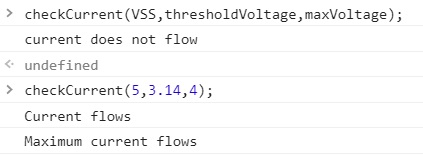The === operator is just like the == operator with the only difference that it not only compares values but their types too. Remember you can find the type of any variable using the typeof operator.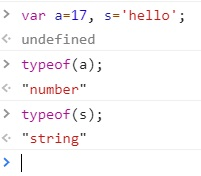```var num = 17;
var str = '17';
console.log(num == str) //true
console.log(num === str) //false
```

Since both num and str have the same values, the == operator gives true. However, they are not essentially the same since they hold the same values but are of different data types hence === returns false.

We can use the Conditional Operator to evaluate the result of an expression like,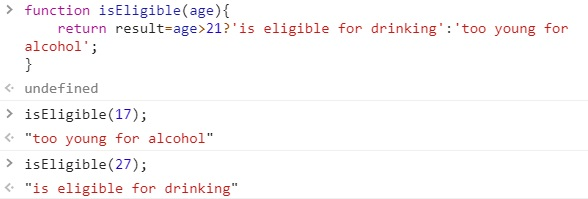### 4) Logical Operators

These operators determine the logic between variables and values. There are &&, || and ! logical operators which stand for logical AND, logical OR and logical NOT.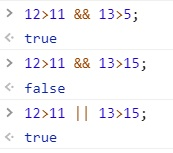The relational and logical operators are often used in combination for the evaluation of certain expressions.

```function evaluate(agg) {

if (agg >= 90)
else if (agg >= 80 && agg < 90)
else if (agg >= 70 && agg < 80)
else if (agg >= 50 && agg < 70)
else
return grade += 'F.You must reappear';

}
```

Languages: » C » C++ » C++ STL » Java » Data Structure » C#.Net » Android » Kotlin » SQL
Web Technologies: » PHP » Python » JavaScript » CSS » Ajax » Node.js » Web programming/HTML
Solved programs: » C » C++ » DS » Java » C#
Aptitude que. & ans.: » C » C++ » Java » DBMS
Interview que. & ans.: » C » Embedded C » Java » SEO » HR
CS Subjects: » CS Basics » O.S. » Networks » DBMS » Embedded Systems » Cloud Computing
» Machine learning » CS Organizations » Linux » DOS
More: » Articles » Puzzles » News/Updates